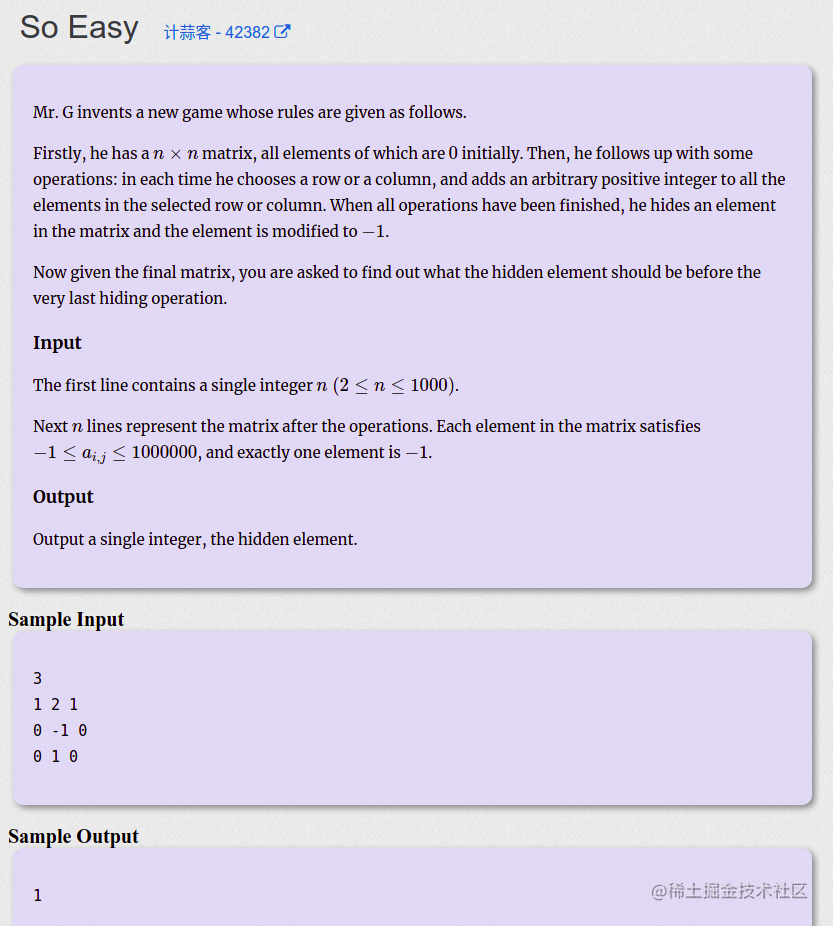# 2019 ICPC 亚洲区域赛银川场 B. So Easy：题解｜刷题打卡

## 一、题目描述：## 二、思路分析：

\begin{aligned} m[row][col] &= m[x][y] + s[row] - s[x] + t[row] - t[y]\\ &= m[x][y] + m[row][y] - m[x][y] + m[x][col] - m[x][y]\\ &= m[row][y] + m[x][col] - m[x][y] \end{aligned}

## 三、AC 代码：

#include <iostream>
using namespace std;
const int maxn = 1005;
int m[maxn][maxn];

int main() {
int n;
scanf("%d", &n);
int row, col, x = 0, y;
for (int i = 0; i < n; ++i) {
for (int j = 0; j < n; ++j) {
scanf("%d", m[i] + j);
if (m[i][j] == -1) {
row = i, col = j;
}
}
}
if (m == -1) {
y = 1;
} else {
y = 0;
}
printf("%d\n", m[x][y] + m[row][y] + m[x][col] - m[x][y]);
}